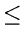Next: 3.12 Programming Assignments Up: 3. Dictionaries Previous: 3.10 To Probe Further

# 3.11 Problems

1.
Consider inserting the keys 10, 22, 31, 4, 15, 28, 17, 88, 59 into a closed hash table of length m = 11 using the primary hashing function h(k) = k mod m. Illustrate the result of inserting these keys using
(a)
Linear probing.
(b)
Quadratic probing with hi(k) = (h(k) + i + 3i2) mod m.
(c)
Double hashing with rehashing function hi(k) = (h(k) + i(1 + k mod(m - 1)) mod m.
2.
A closed hash table of size m is used to store n items, where nm/2.
(a)
Assuming uniform hashing, show that for i = 1, 2,..., n, the probability that the ith insertion requires strictly more than kprobes is at most 2-k.
(b)
Show that for i = 1, 2,..., n, the probability that the ithinsertion requires more than 2log n probes is at most n-2.
3.
Consider a closed hash table with 2n buckets (n > 0), employing the hash function h(x) = x mod 2n. Which of the following rehash strategies would you prefer and why?
(a)
Strategy 1: hi(x) = (h(x) + i) mod 2n.
(b)
Strategy 2: hi(x) = (h(x) + 2i) mod 2n.
4.
Given a set of a maximum of 52 names of 5 characters each and that not more than two names start with the same character, is it possible to find a closed hashing function that operates on a hash table of size 56? Each element of the hash table can be a string of 5 characters. The hashing function should provide for membership test in at most 3 time units. Either exhibit such a hashing function or provide a counter-example. Assume that only upper case alphabetic characters are used in names and that the hashing function can be computed in one time unit. Comparison of strings also takes one time unit.
5.
Consider all two character names with each character being from the set {A, B, C, D, E, F, G, H, I, J}. Design an efficient hashing function such that there would be no collisions on insertion. Assume a hash table of size 115.
6.
Suppose that we are given a key k to search for in a closed hash table with positions 0, 1,..., m - 1, where m is known to be a power of 2. Let h be a hash function. Consider the following search scheme:
1.
Compute i = h(k) and set j = 0.
2.
Probe in position i. Terminate if the required item is found or that position is empty.
3.
Set j = (j + 1)    mod    m and i = (i + j)    mod    m. Return to Step 2.
a.
Does the algorithm examine every table position in the worst case?
b.
Is this linear probing or quadratic probing or double hashing or none?
c.
What is the distinct number of probe sequences?
7.
Given a skip list of 16 elements, compute the (best case) minimum number of probes required for an unsuccessful search in each case below:
(a)
All nodes in the list are level 4 nodes
(b)
There are 14 nodes of level 1 and 2 nodes of level 3
(c)
There is one level 4 node, one level 3 node, and the rest are level 1 nodes
8.
The following results have been experimentally observed by William Pugh in his CACM article on skip lists, while comparing the average case performance of skip lists, splay trees, non-recursive AVL trees, and recursive 2-3 trees. Provide an intuitive justification for each of these:
(a)
The non-recursive AVL tree has the least average case complexity for a dictionary search operation
(b)
The skip list outperforms all other data structures for insertions and deletions
(c)
Splay trees perform better than 2-3 trees for insertionsNext: 3.12 Programming Assignments Up: 3. Dictionaries Previous: 3.10 To Probe Further
eEL,CSA_Dept,IISc,Bangalore